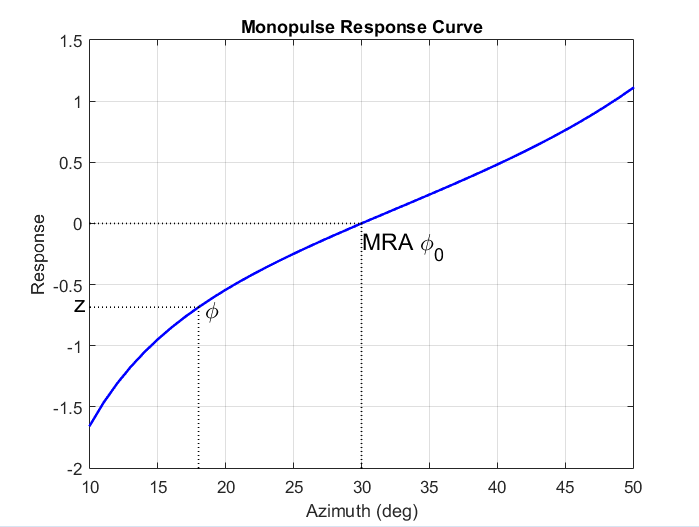# step

System object: phased.SumDifferenceMonopulseTracker
Package: phased

Perform monopulse tracking using ULA

## Syntax

```ESTANG = step(H,X,STANG) ```

## Description

Note

Starting in R2016b, instead of using the `step` method to perform the operation defined by the System object™, you can call the object with arguments, as if it were a function. For example, ```y = step(obj,x)``` and `y = obj(x)` perform equivalent operations.

`ESTANG = step(H,X,STANG)` estimates the incoming direction `ESTANG` of the input signal, `X`, based on an initial guess of the direction.

Note

The object performs an initialization the first time the object is executed. This initialization locks nontunable properties and input specifications, such as dimensions, complexity, and data type of the input data. If you change a nontunable property or an input specification, the System object issues an error. To change nontunable properties or inputs, you must first call the `release` method to unlock the object.

## Input Arguments

 `H` Tracker object of type `phased.SumDifferenceMonopulseTracker`. `X` Input signal, specified as a row vector whose number of columns corresponds to number of channels. You can specify this argument as single or double precision. The size of the first dimension of the input matrix can vary to simulate a changing signal length. A size change can occur, for example, in the case of a pulse waveform with variable pulse repetition frequency. `STANG` Initial guess of the direction, specified as a scalar that represents the broadside angle in degrees. A typical initial guess is the current steering angle. The value of `STANG` is between –90 and 90. The angle is defined in the array's local coordinate system. For details regarding the local coordinate system of the ULA, type `phased.ULA.coordinateSystemInfo`. You can specify this argument as single or double precision.

## Output Arguments

 `ESTANG` Estimate of incoming direction, returned as a scalar that represents the broadside angle in degrees. The value is between –90 and 90. The angle is defined in the array's local coordinate system.

## Examples

expand all

Determine the direction of a target at a 60.1° broadside angle to a ULA starting with an approximate direction of 60°

```array = phased.ULA('NumElements',4); steervec = phased.SteeringVector('SensorArray',array); tracker = phased.SumDifferenceMonopulseTracker('SensorArray',array); x = steervec(tracker.OperatingFrequency,60.1).'; est_dir = tracker(x,60)```
```est_dir = 60.1000 ```

## Algorithms

The sum-and-difference monopulse algorithm is used to the estimate the arrival direction of a narrowband signal impinging upon a uniform linear array (ULA). First, compute the conventional response of an array steered to an arrival direction φ0. For a ULA, the arrival direction is specified by the broadside angle. To specify that the maximum response axis (MRA) point towards the φ0 direction, set the weights to be

`${w}_{s}=\left(1,{e}^{ikd\mathrm{sin}{\varphi }_{0}},{e}^{ik2d\mathrm{sin}{\varphi }_{0}},\dots ,{e}^{ik\left(N-1\right)d\mathrm{sin}{\varphi }_{0}}\right)$`

where d is the element spacing and k = 2π/λ is the wavenumber. An incoming plane wave, coming from any arbitrary direction φ, is represented by

`$v=\left(1,{e}^{ikd\mathrm{sin}\varphi },{e}^{ik2d\mathrm{sin}\varphi },\dots ,{e}^{ik\left(N-1\right)d\mathrm{sin}\varphi }\right)$`

The conventional response of this array to any incoming plane wave is given by ${w}_{s}^{H}v\left(\phi \right)$ and is shown in the polar plot below as the Sum Pattern. The array is designed to steer towards φ0 = 30°.

The second pattern, called the Difference Pattern, is obtained by using phased-reversed weights. The weights are determined by phase-reversing the latter half of the conventional steering vector. For an array with an even number of elements, the phase-reversed weights are

`${w}_{d}=-i\left(1,{e}^{ikd\mathrm{sin}{\varphi }_{0}},{e}^{ik2d\mathrm{sin}{\varphi }_{0}},\dots ,{e}^{ikN/2d\mathrm{sin}{\varphi }_{0}},-{e}^{ik\left(N/2+1\right)d\mathrm{sin}{\varphi }_{0}},\dots ,-{e}^{ik\left(N-1\right)d\mathrm{sin}{\varphi }_{0}}\right)$`

(For an array with an odd number of elements, the middle weight is set to zero). The multiplicative factor –i is used for convenience. The response of the difference array to the incoming vector is

`${w}_{d}^{H}v\left(\phi \right)$`

This figure shows the sum and difference beam patterns of a four-element uniform linear array (ULA) steered 30° from broadside. The array elements are spaced at one-half wavelength. The sum pattern shows that the array has its maximum response at 30° and the difference pattern has a null at 30°.The monopulse response curve is obtained by dividing the difference pattern by the sum pattern and taking the real part.

`$R\left(\phi \right)=Re\left(\frac{{w}_{d}^{H}v\left(\phi \right)}{{w}_{s}^{H}v\left(\phi \right)}\right)$`

To use the monopulse response curve to obtain the arrival angle, φ, of a narrowband signal, x, compute

`$z=Re\left(\frac{{w}_{d}^{H}x}{{w}_{s}^{H}x}\right)$`

and invert the response curve, φ = R-1(z), to obtain φ.

The response curve is not generally single valued and can only be inverted when arrival angles lie within the main lobe where it is single valued This figure shows the monopulse response curve within the main lobe of the four-element ULA array.There are two desirable properties of the monopulse response curve. The first is that it have a steep slope. A steep slope insures robustness against noise. The second property is that the mainlobe be as wide as possible. A steep slope is ensure by a larger array but leads to a smaller mainlobe. You will need to trade off one property with the other.

For further details, see .

## References

 Seliktar, Y. Space-Time Adaptive Monopulse Processing. Ph.D. Thesis. Georgia Institute of Technology, Atlanta, 1998.

 Rhodes, D. Introduction to Monopulse. Dedham, MA: Artech House, 1980.